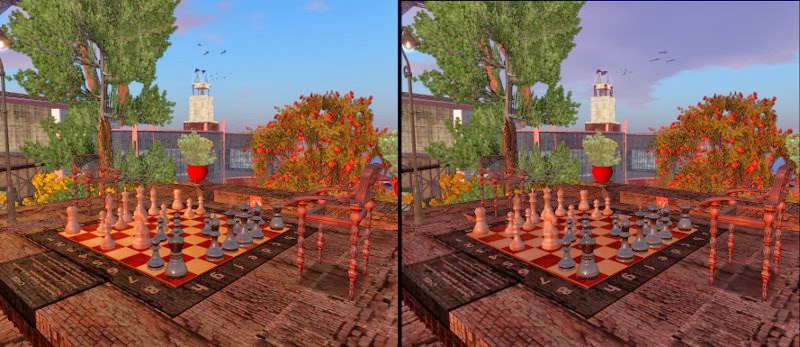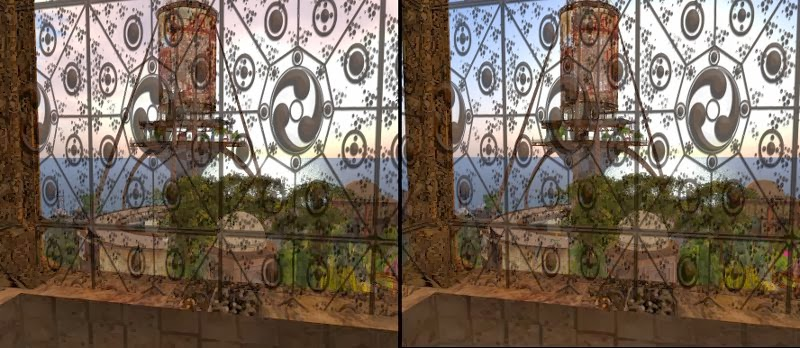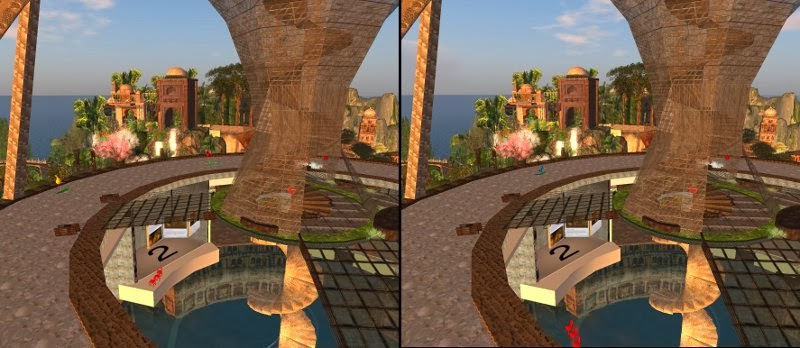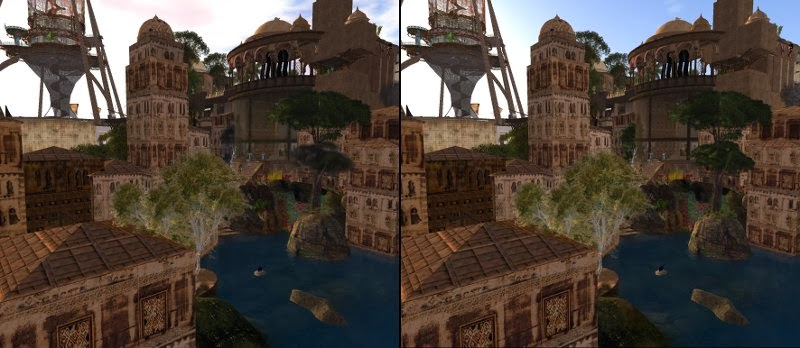## February 15, 2014

### Rotation: a one prim door (more)

In the previous posting on this topic, I mentioned in its Note 2 that a single call to llSetPrimitiveParams() would create a visual flaw in which the hinge wobbles.  The flaw is caused by moving the center of the door in a straight line as it rotates.  It is corrected by moving the center of the door in an arc around the hinge point.

This drop-in replacement code for shiftTo() performs the interpolation.

shiftTo( vector pEnd, rotation rEnd ) {
float swingDuration = 5;            // seconds
integer nSteps      = 50;           // must be positive.

// start at the current location
vector   pStart = llGetPos();
rotation rStart = llGetRot();

// Find the hinge point, get the vector that will swing in an arc
vector pHinge = pStart + lPhingePoint * rStart;
vector vecToCenter = pStart - pHinge;

// Get the angle and axis of the global rotation from start to end
// Since rEnd = rStart * rDelta, left multiply by the inverse

// of rStart to isolate rDelta (note 1)
rotation rDiff = (ZERO_ROTATION / rStart) * rEnd;
float angle = llRot2Angle(rDiff);
vector axis = llRot2Axis(rDiff);

integer k; for ( k=0; k < nSteps; k++ ) {

// for each step, calculate an intermediate rotation difference
// by interpolating the angle and applying it to the fixed axis
float angleDelta = k * angle / (nSteps-1);
rotation rDelta = llAxisAngle2Rot(axis,angleDelta);

// Use the intermediate rotation to rotate the hinge to center vector
// Use the intermediate rotation to turn the object
vector p = pHinge + vecToCenter * rDelta;
rotation r = rStart * rDelta;            // (note 2)

[ PRIM_POSITION, p, PRIM_ROTATION, r]);
llSleep( swingDuration/nSteps );
}
}

As a bonus the door will slowly open and close. (note 3)

__________________
Note 1
I discuss the algebraic manipulation of rotations and inverse rotations in Rotation: Algebra.

Note 2
You may have noticed that in contrast to start_entry() (cf. note 3 in the prior post) the order of rotation multiplication in shiftTo() is reversed, that is, the change in rotation is right multiplied against the starting rotation.

The order is reversed because both of these rotations have values in the global (region) coordinates.

The difference between A*B and B*A is that one ordering corresponds to each rotation occurring in progressively rotated coordinates (as in start_entry()), whereas the other ordering corresponds to all rotations being in the same coordinates (as in shiftTo()).

Note 3
If you wish to add sound effects, I recommend adding calls to llPlaySound() in touch_start().  Place them before and after the appropriate calls to ShiftTo().

[end]

## February 14, 2014

### 3D Vision II: at ACC Alpha

With the permission of Haveit Neox, the creator of the region ACC Alpha, here are a series of convergent (cross-eyed) viewable stereograms of that region.Chess sets are a traditional subject for 3D renderingThrough a screen darkly.  Do visit the TowerThe Tower has many levels and multiple subtextsWalk the streets of the paper villageTour the fine galleriesRelax in a cafe at in the shopping/gallery district

You may these easier to view of you download them and view them at a smaller scale.

My preceding post on stereoscopic imaging my be of interest.

[end]

## February 13, 2014

### Rotation: a one prim door

The simplest method to make a swinging door is to use a box prim that has half of its volume cut away.  The remaining visible section has its local Z axis on an edge.  You can swing the door with

vector doorRot = llEuler2Rot( < 0, delta, 0 > );
llSetRot( doorRot * llGetRot() );

where 'delta' is an angle (radians) that alternates positive and negative on each touch.

However, if you wish to make a Hobbit door from, say, a cylinder, you can still get the edge hinge effect without cutting the prim.   The trick is to move the center of the door as you rotate it so as to keep the global (region) position of the edge in the same place.For this discussion, imagine a cylinder with Z sized down to make a disk.  Orient this with its local Y+ axis upward.  The hinge point will be where the prim local X+ axis intercepts the edge of the cylinder.  The prim will rotate around a vertical axis.

Place this script in the prim.  Then touch the prim.

integer isOpen = 0;
float swingAngleDeg = -80;

vector gPdoorOpen;                  // values in global (region) coordinates
vector gPdoorShut;                  // (note 1)
rotation gRdoorOpen;
rotation gRdoorShut;

vector lPhingePoint;                // value in local (cylinder) coordinates

shiftTo( vector p, rotation r ) {

// There will be a visual problem with this method (note 2)
llSetPrimitiveParams([PRIM_POSITION,p,PRIM_ROTATION,r]);
}

////////////////////////////////////////////////////////////////////
default {
state_entry() {
// The closed position is determined by reset
gPdoorShut = llGetPos();
gRdoorShut = llGetRot();

// The open rotation is an additional local Y axis rotation (note 3)

vector eulerRot = < 0, DEG_TO_RAD*swingAngleDeg, 0 >;
gRdoorOpen = llEuler2Rot(eulerRot) * gRdoorShut;

// Calculate the open position (note 4)
vector size = llGetScale();
lPhingePoint = < size.x/2, 0, 0 >;
gPdoorOpen = gPdoorShut + (lPhingePoint * gRdoorShut)

- (lPhingePoint *     gRdoorOpen);
}

touch_start(integer nTouch) {
isOpen = !isOpen;
if ( isOpen ) {

shiftTo(gPdoorOpen,gRdoorOpen);
} else {
shiftTo(
gPdoorShut,gRdoorShut);
}
}

}

This script will work for any prim size or orientation.  Just remember to reset the script if you change the prim position, rotation, or size.
_____________________________
Note 1:
In this notation, the first letter is l or g and indicates local or global coordinates.  The second letter is P or R and indicates position or rotation.  The remaining letters describe which specific point.

Note 2:
While rotating the center of the prim is moving in a straight line.  The result is that the hinge will wobble.  The correct movement is rotating the prim while moving the center of the prim in an arc about the hinge point.

There are two methods to do this:
• shiftTo() can be a loop that performs a series of small calculated rotations, or
• shiftTo() can create a list of incremental positions along an arc and corresponding rotations and pass this list to llKeyFramedMotion()
However, llKeyFrameMotion() is a flawed implementation that drifts.  You will have to apply corrections when the motion completes, that is, if it completes.  The irksome details are documented in the caveats here.

See this future posting for the loop series fix.

Note 3:
Why A*B and not B*A?  First, for two combined rotations the order matters.  Second, this problem has two rotation: B = the prim rotation with respect to the region when closed (gRdoorShut = llGetPos()), and A = the addition rotation needed to turn the door from closed to open (llEuler2Rot(eulerRot)).

A is a rotation that is expressed in terms of the local coordinates of the prim that has already been rotated by B.   A is specifically a rotation about the prim local Y+ axis (swingAngleDeg).  In this case, where A is relative to B local coordinates, the multiplication order is A*B.

Note 4:
The hinge point has both a global (region coordinates) and local (prim coordinates) position value.  By definition a prim rotation converts a vector in a the prim local coordinates to a vector in the global coordinates relative to the prim center.  Therefore the global position of the hinge point is its rotated local vector added to the prim global position.

When the door is open

gPhingePointOpen = gPdoorOpen + lPhingePoint * gRdoorOpen

and when the door closed is

gPhingePointShut = gPdoorShut + lPhingePoint * gRdoorShut

However the hinge does not change position, so the right hand expressions have the same value.  Now solve the equated right side expressions for gPdoorOpen.  Q.E.D.

[end]

## February 2, 2014

### 3D Vision I: stereoscopic imaging

Human binocular vision allows people to directly perceive depth in their local environment (note 1).   By using pairs of pictures created with slightly different camera viewpoints and letting each eye see only one of these pictures, this innate ability can be tricked into experiencing depth (note 2).

____________
In this pair of images the right image camera viewpoint is slightly to the right of the left image camera viewpoint (look closely at the relationship of the blue arrow to one of the red balls).

If you could 'stare at infinity' in a way that causes your right eye to gaze directly at the right image, and your left eye at the left, then your doubled vision may see a third merged image in the center that has the illusion of depth.   Many people find this to be quite difficult.  We do not have much practice in intentionally diverging our eyes while maintaining a close focal plan.  (note 3)

____________
More people are able to use the 'cross-eyed' viewing technique.  This pair of images has been transposed left to right.

If you can converge (cross) the lines of sight of your eyes while maintaining your focal plane on the images, then your doubled vision may see a third merged image in the center that has the illusion of depth.

____________
All systems that allow you to see 3D in virtual worlds use this technique to create the illusion of depth.  They differ in the methods by which they allow each eye to see one of the images.  They may
• alternately flicker the two images while you wear glasses with alternating shutters (note 4)
• show both images but use goggle optics to diverge and focus your eyes to each image (note 2)
• merge images while tinting each with distinct colors while you wear color screening glasses (note 5)
The optic method allows the images to be placed much closer to the eyes.  By distorting the images with wide view angle and barrel distortion to compensate for the optic effects, you see a more surrounding effect.  (note 2c)

_______________
_______________

Note 1: Beyond several meters distance our binocular vision mechanism becomes ineffective and our depth perception relies on other cues such as parallax, scale consistency, atmospheric degradation, light shading, and memory.  When you watch a conventional movie, all of your sense of depth in the scenes comes from these alternate cues.

Note 2:

Note 3: For diverging ("infinity") viewing it may help to hold up a piece of paper that prevents each eye from seeing the image intended for the other eye.  It may also help to download the images on this post and reduce their size.

[end]

## December 27, 2013

### Generating Bézier Curves

A Bézier curve is a directed path defined by a ordered set of reference points.   It starts at the first point and ends at the last point.  It rarely passes through any of the intermediate points, which instead act as attractors that warp the curve in their direction as the curve passes by them.

For details about this class of curves and several dynamic visualizations, I refer you to the article at this location

http://en.wikipedia.org/wiki/B%C3%A9zier_curve

___________________________________________
This position along a Bézier curve is specified by an independent variable in the range [0,1].  The value '0' is the start of the curve, the value '1' is the end of the curve.

One method of calculating a series of points along a Bézier curve is to use polynomial functions of this variable with coefficients that are linear in the positions of the reference points.  The down side of this method is that you need a different polynomial whenever you change the number of reference points.

My preferred method is recursive (note 1).  Given the ordered list of reference points 'list posList = [ .. vectors...];' and the independent variable 'float s;' in the range [0,1], the calculation proceeds as follows (note 2):

vector getBezierPos( float s ) {
// This method uses the direct progressive linear interpolation definition
// of an n-th order bezier, instead of the clumbersome formulae.
list q = posList;
integer nq;
while ( (nq=llGetListLength(q)) > 1 ) {
list q2 = [];
integer k; for ( k=1; k < nq; k++ ) {

vector p1 = llList2Vector(q,k-1);
vector p2 = llList2Vector(q,k);
q2 += (1-s)* p1 + s * p2;
}
q = q2;
}
return llList2Vector(q,0);
}

In the Wikipedia reference, find the dynamic visualization that demonstrates the construction of a Bezier curve as a progression of linear interpolations.  My implementation of getBezierPos() exactly follows that demonstration.

___________________________________________
To elucidate the selection of values of 's', I will add here the function which rezzes prims along the Bézier curve.

integer nDot = 20;
string dotName = "dot";

generateBezierDots() {
// The Bézier parameters is a variable in the range [0,1].  Divide this
// interval into nDot segments. Rez dots at the mid point of these segments.
float step = 0;
if ( nDot > 0 )  step = 1.0 / nDot;

integer k; for ( k=1; k <= nDot; k++ ) {
vector p = getBezierPos( step * (k - 0.5) );
llRezObject(dotName, p, ZERO_VECTOR, ZERO_ROTATION, 0 );
}
}

___________________________________________
In my full implementation (note 3) of this script, I use llSensor() to find a series of reference markers.  The sensor() event makes note of the positions and descriptions of these prims, the description fields of the markers are used to sort the data, and the ordered position are stored in posList.

Desdemona

_____________________________________________
Note 1
Purists will note that I did not strictly use a recursive function.  Ultra purists will complain that said function should have been tail recursive.  I will leave it to them to post the pure implementation on their blogs and let them cope with a stack usage that that is quadratic in the number of reference points and with LSL not supporting tail recursion.

Note 2
If you want a vector tangent to the curve, in getBezierPos() catch the value"p2-p1" when nq is 2.

Note 3
If you would like a copy of my full implementation, come to my vast land empire of 512 m-sq and pick up a copy from my vendor titled "Simple Bezier Curve".  You will get a combo object (mod/copy/trans, including scripts) that includes 4 green reference markers.  Rez this and touch the blue sphere.  Voila, 20 pink dots rezzed along the curve.

Move the reference markers around and touch the blue sphere again.  Delete reference markers or shift copy more reference markers as you wish, however, make sure they all have distinct descriptions that will order them as you wish.

The vendor is here:

http://maps.secondlife.com/secondlife/Pomponio/191/155/59

[end]